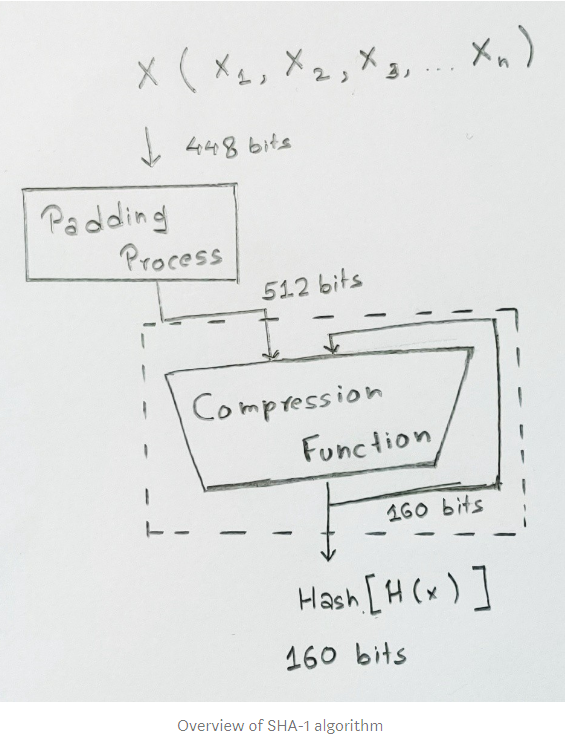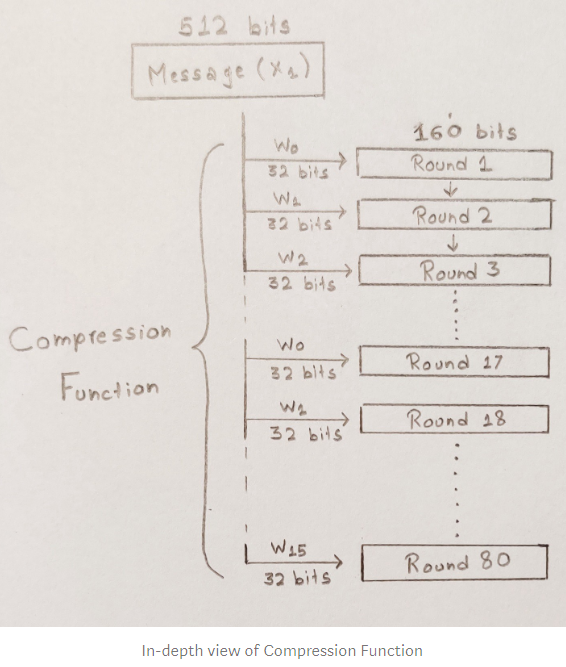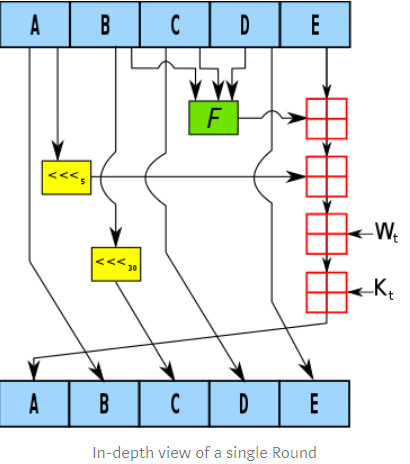• No products in the cart.

# Breaking Down: SHA-1 Algorithm

I haven’t been blogging for the past month or so and to be true the reason for that was that I totally out of content. You could say I simply ran out of gas, so I took this time off and went back to the basics studying day in and day out. It involved a lot of things, secure coding, cloud security, docker and docker security, hashing and cryptography. So now, that I have been focused on these things you can look forward to articles on these topics. You can definitely ping me if there is something, in particular, you want me to write about and explain it to you in detail.

Let’s get back to what we are here for, Secure Hashing Algorithm, aka SHA, is a hashing technique that has been used for various reasons in tech ranging from checksum, file integration verification, password verification etc. All the hashing techniques that are present have these two prominent features i.e. the output of these hash functions will be of fixed length it can vary from a hash function to the another but for a particular hash function, the length of the hash won’t ever change. Hash functions are also compression functions hence their output is mostly referred to as digests.

The reason I am writing this article is that even though most of the security community is aware of these algorithms and use them as part of libraries they import but most of them are actually unaware of how exactly a hash is a non-invertible and why it cannot be reverse-engineered. Having an in-depth knowledge of how the hash techniques work and why one hashing algorithm is better than the other helps a lot in the field of cybersecurity and gives you the understanding of which algorithms to use rather than just downloading libraries and running them in our programs.

# Let’s begin!

Before we get deep into understanding SHA-1 and its algorithm. Firstly we need to know the required rule or properties that every hashing algorithm must follow and adhere to. There are two such rules, first, the original message should not derivable from the message digest that was generated and the second, two different messages should never have the same message digest. As long we understand these we will understand in the next steps why such a complex and irreversible process are used in hashing algorithms.

Well, first of all, let’s have a birds-eye view of what the hashing algorithm is all about.The leftmost part of the image is our message that we want to be hashed, then we carry out the hashing function on our message, to receive the hash in this particular case it is of 160 bits.Let’s have a more in-depth view of the image on the left. First, we break our message into ’n’ number of parts which we are depicting as X, each of size of 448 bits and then we add 64 bits of padding to each of them which converts their total length to 512 bits. These 512 bits are then put in the compression function along with the 160 bits of compressed output, for the first time we carry this out we have a predefined value for the 160 bit value.

This process keeps on going on ’n’ number of times over and over again till the last 160 bit of the message is produced and that is the hash depicted as H(x)

# Compression Function

Now let’s take a deeper look at the compression function in the above image. The compression function in itself has a total of 80 rounds in it and keep it in mind that these 80 rounds take place for each part of the image that when padded sums up to the 512 bits each.Now, the next part is that these 512 bits are further divided into 16 parts each of 32 bits and these are marked from M1 to M16.

So, currently, we have 80 rounds of computation left to be carried out, with the 512 bits message that we have divided into 16 parts each of 32 bits.

The problem is that we have only 16 distinct parts of the message and 80 rounds of computation hence we repeat these 16 parts over and over for five times in the exact sequence they are in M1 to M16.

In the beginning, we have 160 bits input, we break it down into 5 parts which we name to be A, B, C, D & E. The initial values for A, B, C, D & E are mentioned below

```A = 0x67452301
B = 0xEFCDAB89
D = 0x10325476
E = 0xC3D2E1F0```

Once, we break them down into these 5 parts then we again have a set of complex procedures that we carry out in each of these rounds. That is the base core and reason for the complexity of the SHA-1 algorithm.We can see that I have mentioned F(t), W(t) & K(t). We already know about W(t), these are the set of 32 bits, part of the 512 bits message but we are still unaware of F(t) and K(t). These are unique set of functions and values that are used to compute the hash and they remain constant for every 20 rounds i.e. they change 4 times while 80 rounds of computations take place. For every 20 rounds, F(t) and K(t) are constant they have a set of predefined values and function description which remains common depicted below.

```Rounds 1-20
f(1) = (B and C) or ((not B) and D)
k(1) = 0x5A827999Rounds 21-40
f(2) = B xor C xor D
k(2) = 0x6ED9EBA1Rounds 41-60
f(3) = (B and C) or (B and D) or (C and )
k(3) = 0x8F1BBCDCRounds 61-80
f(4) = B xor C xor D
k(4) = 0xCA62C1D6```

# Conclusion

The SHA-1 algorithm is complex no doubt, and the bigger news is that it is not used anymore as it has been cracked and is deemed not be safe anymore. So, to have an overview of the whole things let me explain it again in layman term in a paragraph.

The message that needs to be hashed is broken down into 448 bits of pieces and another 64 bits is padded to it which acts a message block going ahead. This message block is then again broken down into 16 parts, each of 32 bits and then passed into the compression function. The compression function consists of 80 rounds of computation. These 16 parts of message block is fed into these rounds and it repeats in a sequential manner after every 16 round. The 160 bit which also enters the compression function is broken down into 5 parts ( A, B, C, D & E ) where again a set of instructions are carried out where we apply the F(t) on B,C & D and perform modulus operation with the message block and then with the K(t), the key pair. There are 4 distinct F(t) and K(t) and these are predefined for the SHA-1 algorithm and remains same for 20 rounds of computations. After 80 such rounds the 160 bits of output is again poured back into the compression function but this time the second 448 bits of the message and it continues till the last bits of the original message is computed upon and so we get the 160 bits output which is the hash for the original message we passed.

So that’s the short version of the whole functioning of the SHA-1 algorithm.

## Credits:

1. https://www.youtube.com/watch?v=plxMklEvlCU — I referred to this video for the basic understanding of SHA-1
2. https://en.wikipedia.org/wiki/SHA-1 — The Wikipedia article on SHA-1

CyberSec professional, crazy about tinkering with computers.

I am a bug hunter and specialise in helping companies and organisations, by finding bugs in their web / mobile applications and help them solve it. Freelancing in the field of networking and cybersecurity. Spend my days working on Kali. Well-versed programmer in C, C++, Bash Script and JAVA language.

E-mail : [email protected]

The article has been originally published at: https://medium.com/bugbountywriteup/breaking-down-sha-1-algorithm-c152ed353de2

November 20, 2019
Subscribe
Notify ofThis site uses Akismet to reduce spam. Learn how your comment data is processed.

1 Comment
Inline Feedbacks